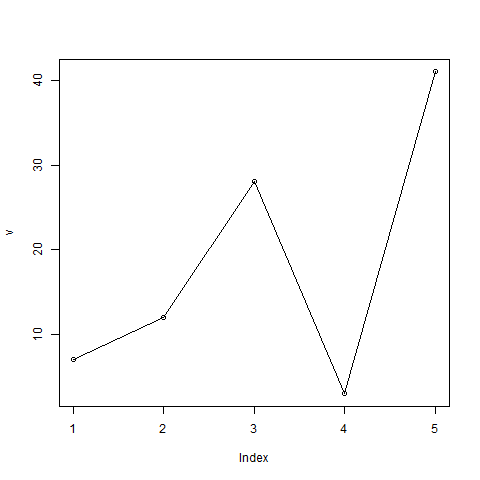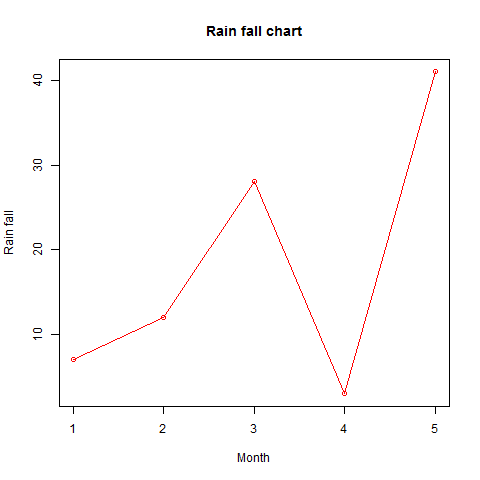R在线运行折线图

折线图

R语言中的plot()函数用于创建折线图。

语法

plot(v,type,col,xlab,ylab)

• v是包含数值的向量。

• 类型采用值“p”仅绘制点，“l”仅绘制线和“o”绘制点和线。

• xlab是x轴的标签。

• ylab是y轴的标签。

• main是图表的标题。

• col用于给点和线的颜色。

例

# Create the data for the chart.
v <- c(7,12,28,3,41)

# Give the chart file a name.
png(file = "line_chart.jpg")

# Plot the bar chart.
plot(v,type = "o")

# Save the file.
dev.off()折线图标题，颜色和标签

例

# Create the data for the chart.
v <- c(7,12,28,3,41)

# Give the chart file a name.
png(file = "line_chart_label_colored.jpg")

# Plot the bar chart.
plot(v,type = "o", col = "red", xlab = "Month", ylab = "Rain fall",
main = "Rain fall chart")

# Save the file.
dev.off()多线型折线图

# Create the data for the chart.
v <- c(7,12,28,3,41)
t <- c(14,7,6,19,3)

# Give the chart file a name.
png(file = "line_chart_2_lines.jpg")

# Plot the bar chart.
plot(v,type = "o",col = "red", xlab = "Month", ylab = "Rain fall",
main = "Rain fall chart")

lines(t, type = "o", col = "blue")

# Save the file.
dev.off()JSRUN提供的R在线运行，R 在线运行工具，基于linux操作系统环境提供线上编译和线上运行，具有运行快速，运行结果与常用开发、生产环境保持一致的特点。

• 00:23